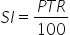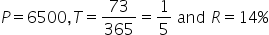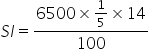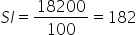Maths-
General
Easy

Question

# Find the simple interest on Rs. 6500 at 14% per annum for 73 days?Hint:

## The correct answer is: 182 Rupees

### Complete step by step solution:We calculate simple interest by the formula,where P is Principal amount, T is number of years and R is rate of interestHere, we haveOn substituting the known values we get,On further simplifications, we haverupees.Thus, SI = 182 Rupees.#### With Turito Foundation.#### Get an Expert Advice From Turito.